# 算法基础（一）| 快速排序和归并排序详解

• 2022 年 9 月 11 日
山东
• 本文字数：2114 字

阅读完需：约 7 分钟

⭐写在前面的话：本系列文章旨在复习算法刷题中常用的基础算法与数据结构，配以详细的图例解释，总结相应的代码模板，同时结合例题以达到最佳的学习效果。本专栏面向算法零基础但有一定的 C++基础的学习者。若 C++基础不牢固，可参考：10min快速回顾C++语法，进行语法复习。

🔥本文已收录于算法基础系列专栏： 算法基础教程 免费订阅，持续更新。## 快速排序

### 算法详解

• 确定分界点

• 常用分界点：取左边界 q[l],取中间值 q[(1+r)/2],取右边界 q[r],随机值。

• 调整区间

• 保证左边数都小于等于 x，右边数大于等于 x 即可。

•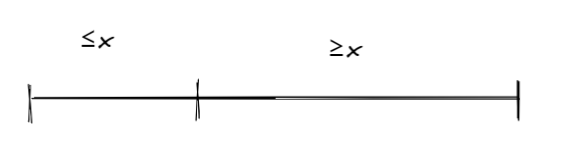• 递归处理左右两端

• 左边排好序，右边排好序。

1. 双数组法（比较耗费空间）

2. 再开两个数组，分别是 a[],b[].

3. 然后对于原数组 q，如果 q[i] ≤ x，则将 x 存入 a[]中，否则存入 b[]中。

4. 最后先将 a[]存入 q 中，再将 b[]存入数中。

5. 双指针法

6. 采用双指针的思想。设置哨兵 x 进行比较。

7.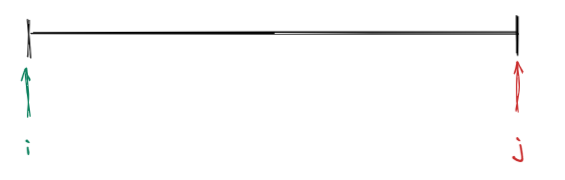8. 让 i，j 向中间移动，如果 i ≤ x，则继续移动，否则等待交换，如果 j ≥ x ，则继续移动，否则等待交换。当 i 和 j 都等待交换的时候，交换 ij，然后继续移动。直到 i 大于为止。

9.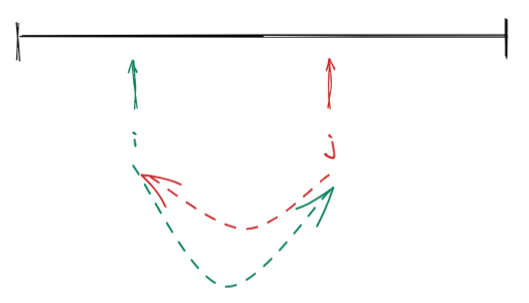10.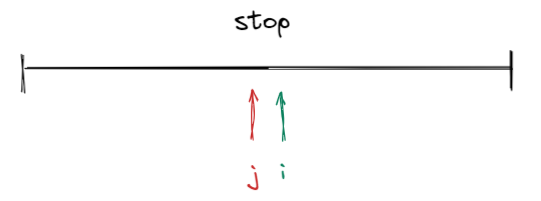### 例题：快速排序

1≤n≤100000

53 1 2 4 5

1 2 3 4 5

### 算法模板

#include <bits/stdc++.h>using namespace std;const int N = 1e6 +10;int q[N];void quick_sort(int q[], int l, int r){    if (l >= r) return;    //如果数组中就一个数，直接返回    int x = q[l], i = l - 1, j = r + 1;    //随机取数，注意这里i要在左边界左边，j要在右边界右边，是因为采用dowhile循环，开场会先执行一次。    while (i < j)    {        do i ++ ; while (q[i] < x);        do j -- ; while (q[j] > x);        if (i < j) swap(q[i], q[j]);        //当两侧都停下后，交换位置即可。    }    //递归地调用函数，排序左右两边。同时注意这里可能存在问题。    quick_sort(q, l, j);    quick_sort(q, j + 1, r);}int main(){    int n;    scanf("%d", &n);    for (int i = 0; i < n; i ++ ) scanf("%d", &q[i]);    quick_sort(q, 0, n - 1);    for (int i = 0; i < n; i ++ ) printf("%d ", q[i]);    return 0;}

    int x = q[l], i = l - 1, j = r + 1;    while (i < j)    {        do i ++ ; while (q[i] < x);        do j -- ; while (q[j] > x);        if (i < j) swap(q[i], q[j]);        //假设是[1,2] x = 1，多轮交换过后一直是[0,1]，死循环就出不来了。    }    同时注意这里可能存在问题。    quick_sort(q, l, j);//如果这里是j的话，x就一定不能取到q[r]    //quick_sort(q, l, i - 1);如果这里是i的话，x就一定不能取到q[l]    //quick_sort(q, i, r);    quick_sort(q, j + 1, r);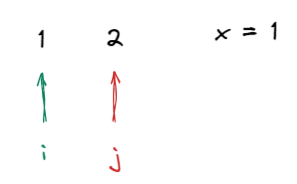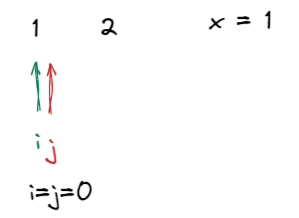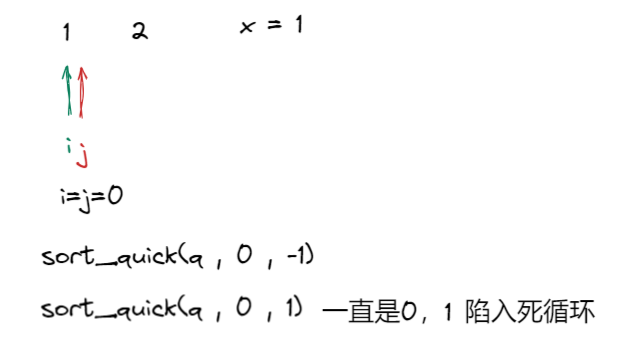## 归并排序

### 算法详解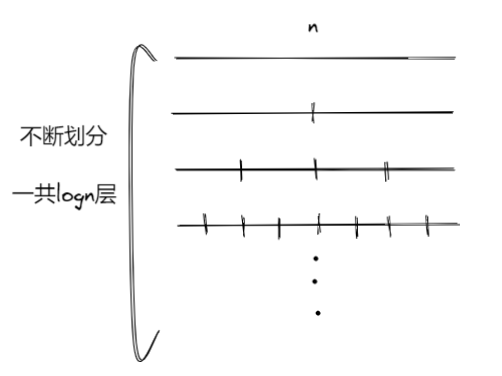• 确定分界点。mid = (1+r)/2;

• 递归排序 left 和 right。

• 合二为一（重点）。合成一个有序的数组。

• 合并的方法：双指针法。

•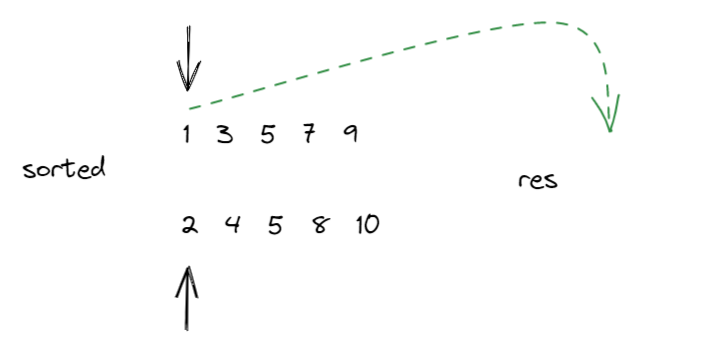•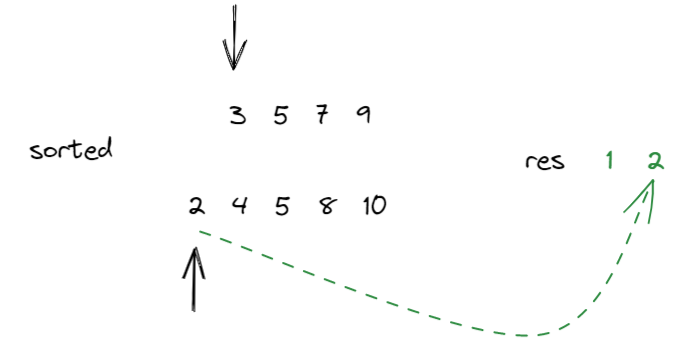•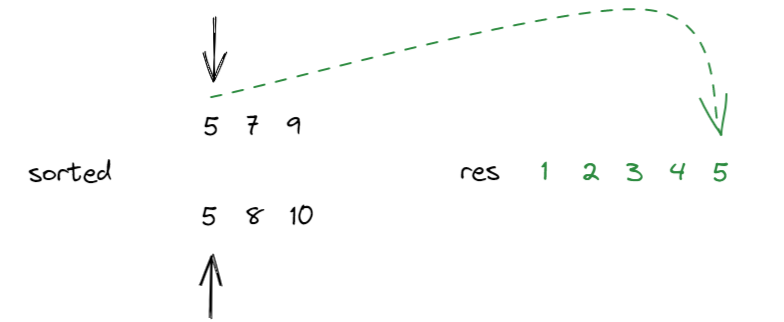• 由于归并排序是稳定的，因此在两数相同的时候可以把第一个数字移动到尾部。

•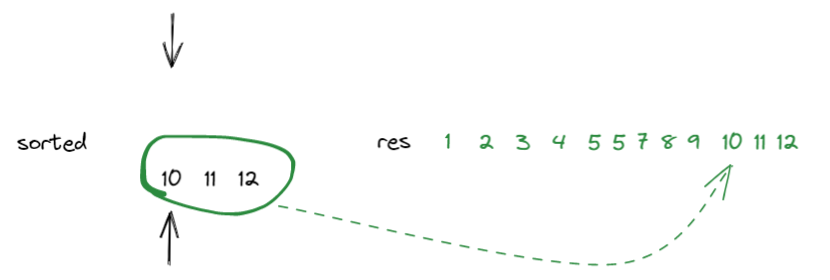### 例题：归并排序

1≤n≤100000

53 1 2 4 5

1 2 3 4 5

### 算法模板

#include <iostream>using namespace std;const int N = 1e5 + 10;int a[N], tmp[N];void merge_sort(int q[], int l, int r){    if (l >= r) return;    int mid = l + r >> 1;    //取中间的位置    //分别归并排序左右两侧，进行排序    merge_sort(q, l, mid), merge_sort(q, mid + 1, r);    //=========归并的过程===========    //k表示已经归并的数，i为指向左半边序列的起点，j为指向右半边序列的起点。    int k = 0, i = l, j = mid + 1;    while (i <= mid && j <= r)        //进行判断，每次把小的那部分放在当前位置上。        if (q[i] <= q[j]) tmp[k ++ ] = q[i ++ ];        else tmp[k ++ ] = q[j ++ ];    //如果左右两边没有循环完的话，贴在数组最后    while (i <= mid) tmp[k ++ ] = q[i ++ ];    while (j <= r) tmp[k ++ ] = q[j ++ ];    //存回q数组中    for (i = l, j = 0; i <= r; i ++, j ++ ) q[i] = tmp[j];}int main(){    int n;    scanf("%d", &n);    for (int i = 0; i < n; i ++ ) scanf("%d", &a[i]);    merge_sort(a, 0, n - 1);    for (int i = 0; i < n; i ++ ) printf("%d ", a[i]);    return 0;}## 评论